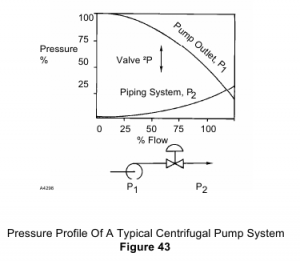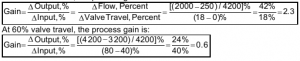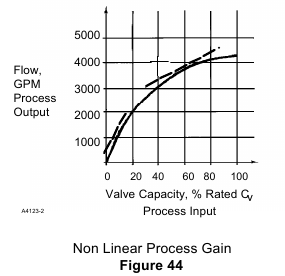# Control Valve – System Characteristics

Typical Pump And Piping System – The objective of flow characterization is to select a valve gain curve that will match with, or compensate for, the gain of the process system. To illustrate, consider the typical pump and piping system that is shown in Figure 43. This system includes a fixed-speed, centrifugal pump. A primary characteristic of a centrifugal pump is that the pressure at the pump outlet (P1) decreases as the flow rate increases. Because of piping friction, the downstream pressure (P 2 ) increases as the flow rate increases. The combined effect of the pump curve and the system curve is that as flow increases, the pressure drop across the valve decreases.Combined Process And Valve Gain – For the purpose of selecting an appropriate valve characteristic, the system characteristics, or process gain, can be analyzed. The process gain will be defined in terms of the change in flow, or GPM (the process output) relative to the change in valve capacity, (the process input). Figure 44 shows a plot of the process gain.

Calculating Gain – Refer to Figure 44 and note that the process gain is defined by the slope of the curve at any operating point. For purposes of illustration, the process gain will be calculated at 10 percent travel and at 60 percent travel. At 10% valve travel, the process gain is:Non-Linear Gain – The plot and the calculations above illustrate a process that is non-linear. If this system were to be operated over a broad range of flow conditions, the following would occur:
• At low flow rates, a given change in the input signal (controller output to the actuator) will cause a large change in flow.
• At high flow rates, the same amount of change in the input signal (controller output to the actuator) will cause a very small change in flow rate.

Controllability And Operating Range – Non-linear process gain can make it difficult or impossible to tune the controller for stable control, unless the system will be operated over a fairly narrow range of conditions.

Non-Linear Process Gain And Pressure Drop – As is often the case, the non-linear process gain that has been demonstrated in this example is the result of a decreasing pressure drop with increasing flow rate.